# Marginal evidence for cosmic acceleration from Type Ia supernovae

## Abstract

The ‘standard’ model of cosmology is founded on the basis that the expansion rate of the universe is accelerating at present — as was inferred originally from the Hubble diagram of Type Ia supernovae. There exists now a much bigger database of supernovae so we can perform rigorous statistical tests to check whether these ‘standardisable candles’ indeed indicate cosmic acceleration. Taking account of the empirical procedure by which corrections are made to their absolute magnitudes to allow for the varying shape of the light curve and extinction by dust, we find, rather surprisingly, that the data are still quite consistent with a constant rate of expansion.

## Introduction

In the late 1990’s, studies of Type Ia supernovae (SN Ia) showed that the expansion rate of the universe appears to be accelerating as if dominated by a cosmological constant1,2,3. Since then supernova cosmology has developed rapidly as an important probe of ‘dark energy’. Empirical corrections are made to reduce the scatter in the observed magnitudes by exploiting the observed (anti) correlation between the peak luminosity and the light curve width and the colour4,5. Other such correlations have since been found e.g. with the host galaxy mass6 and metallicity7. Cosmological parameters are then fitted, along with the parameters determining the light curves, by simple χ2 minimisation1,8,9,10,11. This method has a number of pitfalls as has been emphasised earlier12,13.

With ever increasing precision and size of SN Ia datasets, it is important to also improve the statistical analysis of the data. To accomodate model comparison, previous work14,15,16 has introduced likelihood maximisation. In this work we present an improved maximum likelihood analysis, finding rather different results.

## Supernova Cosmology

There are several approaches to making SN Ia ‘standardiseable candles’. The different philosophies lead to mildly different results but the overall picture seems consistent17. In this paper we adopt the widely used approach of ‘Spectral Adaptive Lightcurve Template 2′ (SALT2)18,19 wherein the SN Ia are standardised by fitting their light curve to an empirical template, and the parameters of this fit are used in the cosmological analysis. (A more comprehensive statistical model of light curves spanning optical through near-infrared data has subsequently been constructed in a hierarchical Bayesian framework20). Every SN Ia is assigned three parameters, one being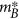, the apparent magnitude at maximum (in the rest frame ‘B-band’), while the other two describe the light curve shape and colour corrections: x1 and c. The distance modulus is then taken to be: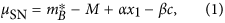where M is the absolute magnitude, and α and β are assumed to be constants for all SN Ia. These global constants are fitted along with the cosmological parameters. The physical mechanism(s) which give rise to the correlations that underlie these corrections remain uncertain21,22. The SN Ia distance modulus is then compared to the expectation in the standard ΛCDM cosmological model: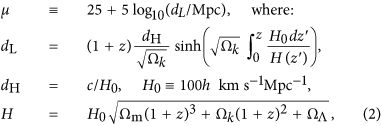where dL, dH, H are the luminosity distance, Hubble distance and Hubble parameter respectively, and Ωm, ΩΛ, Ωk are the matter, cosmological constant and curvature density in units of the critical density3. There is a degeneracy between H0 and M0 so we fix the value of the Hubble parameter today to h = 0.7 which is consistent with independent measurements.

## Maximum Likelihood Estimators

To find the maximum likelihood estimator (MLE) from the data, we must define the appropriate likelihood: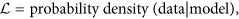i.e. we have to first specify our model of the data. For a given SN Ia, the true data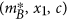are drawn from some global distribution. These values are contaminated by various sources of noise, yielding the observed values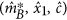. Assuming the SALT2 model is correct, only the true values obey equation (1). However when the experimental uncertainty is of the same order as the intrinsic variance as in the present case, the observed value is not a good estimate of the true value. Parameterising the cosmological model by θ, the likelihood function can be written as13: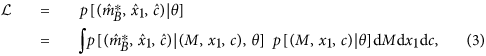which shows explicitly where the experimental uncertainties enter (first factor) and where the variances of the intrinsic distributions enter (second factor).

Having a theoretically well-motivated distribution for the light curve parameters would be helpful, however this is not available. For simplicity we adopt global, independent gaussian distributions for all parameters, M, x1 and c (see Fig. 1), i.e. model their probability density as: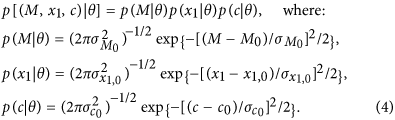All 6 free parameters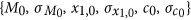are fitted along with the cosmological parameters and we include them in θ. Introducing the vectors Y = {M1, x11, c1, … MN, x1N, cN}, the zero-points Y0, and the matrix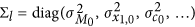, the probability density of the true parameters writes: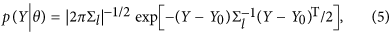where |…| denotes the determinant of a matrix. What remains is to specify the model of uncertainties on the data. Introducing another set of vectors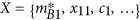, the observed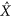, and the estimated experimental covariance matrix Σd (including both statistical and systematic errors), the probability density of the data given some set of true parameters is: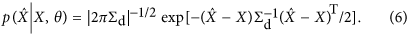To combine the exponentials we introduce the vector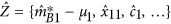and the block diagonal matrix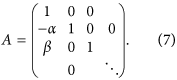With these, we have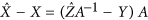and so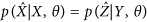. The likelihood is then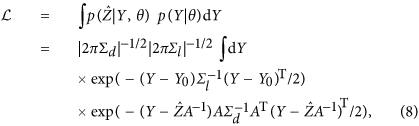which can be integrated analytically to obtain: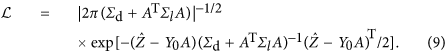This is the likelihood (equation (3)) for the simple model of equation (4), and the quantity which we maximise in order to derive confidence limits. The 10 parameters we fit are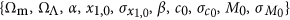. We stress that it is necessary to consider all of these together and Ωm and ΩΛ have no special status in this regard. The advantage of our method is that we get a goodness-of-fit statistic in the likelihood which can be used to compare models or judge whether a particular model is a good fit. Note that the model is not just the cosmology, but includes modelling the distributions of x1 and c.

With this MLE, we can construct a confidence region in the 10-dimensional parameter space by defining its boundary as one of constant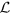. So long as we do not cross a boundary in parameter space, this volume will asymptotically have the coverage probability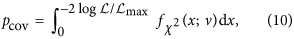where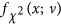is the pdf of a chi-squared random variable with ν degrees of freedom, and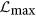is the maximum likelihood.

To eliminate the so-called ‘nuisance parameters’, we set similar bounds on the profile likelihood. Writing the interesting parameters as θ and nuisance parameters as ϕ, the profile likelihood is defined as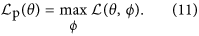We substituteby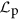in equation (10) in order to construct confidence regions in this lower dimensional space; ν is now the dimension of the remaining parameter space. Looking at the Ωm − ΩΛ plane, we have for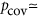{0.68 (“1σ”), 0.95 (“2σ”), 0.997 (“3σ”)}, the values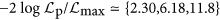respectively.

### Comparison to other methods

It is illuminating to relate our work to previously used methods in SN Ia analyses. One method14 maximises a likelihood, which is written in the case of uncorrelated magnitudes as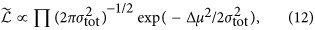so it integrates over μSN to unity and can be used for model comparison. From Equation (3) we see that this corresponds to assuming flat distributions for x1 and c. However the actual distributions of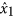andare close to gaussian, as seen in Fig. 1. Moreover although this likelihood apparently integrates to unity, it accounts for only thedata. Integration over the x1, c data demands compact support for the flat distributions so the normalisation of the likelihood becomes arbitrary, making model comparison tricky.

More commonly used1,8 is the ‘constrained χ2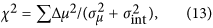but this cannot be used to compare models, since it is tuned to be 1 per degree of freedom for the ΛCDM model by adjusting an arbitrary error σint added to each data point. This has been criticised12,13, nevertheless the method continues to be widely used and the results presented without emphasising that it is intended only for parameter estimation for the assumed (ΛCDM) model, rather than determining if this is indeed the best model.

## Analysis of JLA catalogue

We focus on the Joint Lightcurve Analysis (JLA) catalogue11. (All data used are available on http://supernovae.in2p3.fr/sdss_snls_jla/ReadMe.html — we use the covmat_v6.) As shown already in Fig. 1, the distributions of the light curve fit parametersandare well modelled as gaussians. Maximisation of the likelihood under specific constraints is summarised in Table 1 and the profile likelihood contours in the Ωm − ΩΛ plane are shown in Fig. 2. In Fig. 3 we compare the measured distance modulus,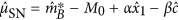with its expected value in two models: ‘ΛCDM’ is the best fit (Table 1) accelerating universe, while ‘Milne’ is an universe expanding with constant velocity. The error bars are the square root of the diagonal elements of Σl + AT−1ΣdA−1 so include both experimental uncertainties and intrinsic dispersion. We show also the residuals with respect to the Milne model (which has been raised to take into account the change in M0).

To assess how well our Gaussian model for the latent variables describes the data, we show the ‘pull’ distribution in Fig. 4. These are defined as the normalised, decorrelated residuals of the data,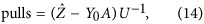where U is the upper triangular Cholesky factor of the covariance matrix Σd + ATΣlA. Performing a K-S test, comparing the pull distribution to a unit variance gaussian gives a p-value of 0.1389.

To check the validity of our method and approximations, we do a Monte Carlo simulation of experimental outcomes from a model with parameters matching our best fit (see Table 1). Figure 5 shows the distribution of, which is just as is expected.

## Discussion

That the SN Ia Hubble diagram appears consistent with an uniform rate of expansion has been noted earlier16,23,24,25. We have confirmed this by a statistically principled analysis, using the JLA catalogue of 740 SN Ia processed by the SALT2 method. We find marginal (i.e.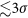) evidence for the widely accepted claim that the expansion of the universe is presently accelerating3.

The Bayesian equivalent of this method (a “Bayesian Hierarchical Model”) has been presented elsewhere13 and has recently been applied to the same dataset, finding results consistent with ours26. We note that a Bayesian consistency test27 has been applied (albeit using the flawed ‘likelihood’ (equation 12) and ‘constrained χ2’ (equation 13) methods) to determine the consistency between the SN Ia data sets acquired with different telescopes28. These authors do find inconsistencies in the UNION2 catalogue but none in JLA. This test had been applied earlier to the UNION2.1 compilation finding no contamination, but those authors29 fixed the light curve fit ‘nuisance’ parameters, so their result is inconclusive. Including a ‘mass step’ correction for the host galaxies of SN Ia11 has little effect.

While our gaussian model (4) is not perfect, it appears to be an adequate first step towards understanding SN Ia standardisation. One might be concerned that various selection effects (e.g. Malmquist bias) affect the data. Such effects may not be amenable to our approximate method and are better addressed in a Bayesian approach26. We are concerned here solely with performing the analysis in a statistically sound manner to highlight the different conclusion from previous analyses11 of the same data.

Whether the expansion rate is accelerating or not is a kinematic test and it is only for ease of comparison with previous results that we have chosen to show the impact of doing the correct statistical analysis in the ΛCDM framework. In particular the ‘Milne model’ refers here to an equation of state p = −ρ/3 and should not be taken to mean an empty universe. For example the deceleration due to gravity may be countered by bulk viscosity associated with the formation of structure, resulting in expansion at approximately constant velocity even in an universe containing matter but no dark energy30. Such a cosmology is not prima facie in conflict with observations of the angular scale of fluctuations in the cosmic microwave background or of baryonic acoustic oscillations, although this does require further investigation. In any case, both of these are geometric rather than dynamical measures and do not provide compelling direct evidence for a cosmological constant — rather its value is inferred from the assumed ‘cosmic sum rule’: ΩΛ = 1 − Ωm + Ωk. This would be altered if e.g. an additional term due to the ‘back reaction’ of inhomogeneities is included in the Friedmann equations31.

The CODEX experiment on the European Extremely Large Telescope will aim to measure the ‘redshift drift’ over a 10–15 year period to determine whether the expansion rate is really accelerating32.

## Methods: Confidence ellipsoids

The confidence ellipsoid is the collection of points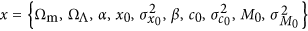, which obey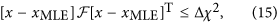where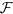is a symmetric matrix and xMLE is the MLE. The enclosed volume is a confidence region with coverage probability corresponding with high precision to the value obtained from Equation (10). The eigenvectors ofare then the principal axes of the ellipsoid, and the eigenvalues are the inverse squares of the lengths of the principal axes. We approximate this matrix with the sample covariance from the MC of section 3 as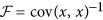.

To make reading the matrix of eigenvectors easier, we round all numbers to 0.1. Thus, we get the following approximate eigenvectors of, in columns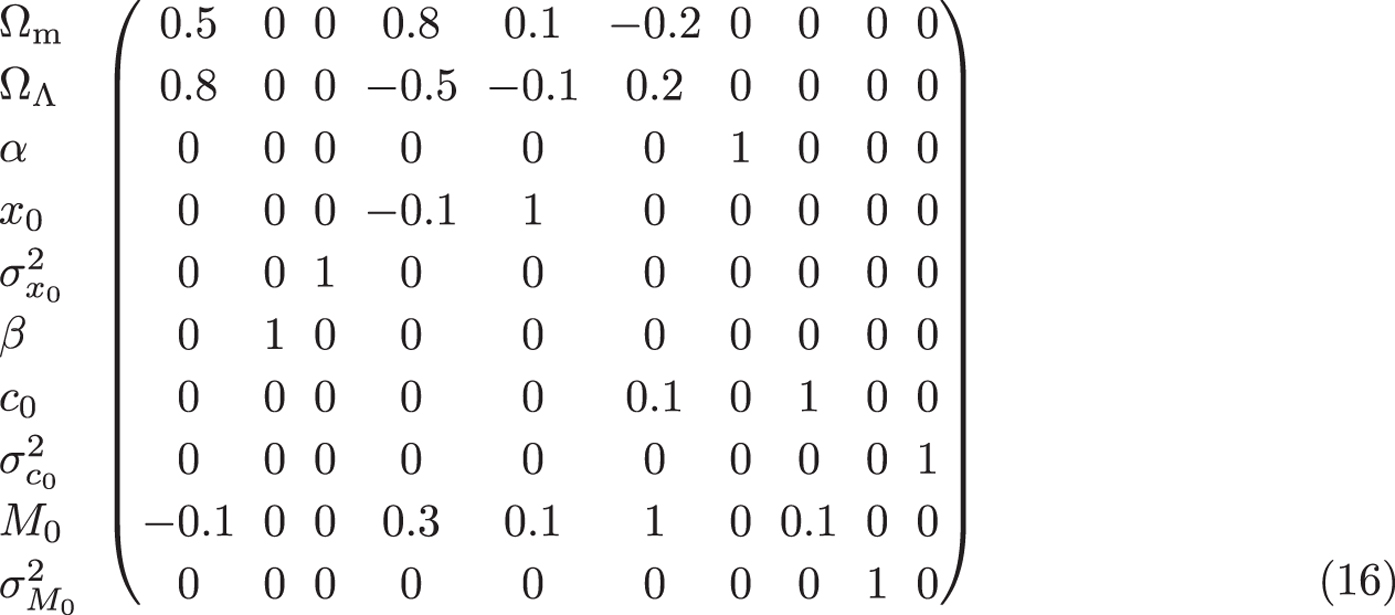with respective lengths of semi-axes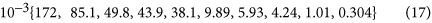We also list the rounded correlation matrix,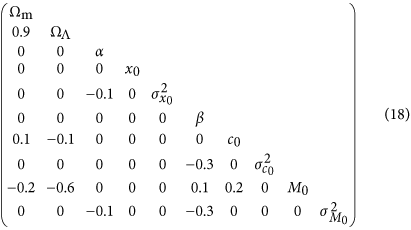We see that the only pronounced correlations are between Ωm, ΩΛ and M0. This is also apparent from Table 1.

### Code Availability

The code and data used in the analysis are available at: http://dx.doi.org/10.5281/zenodo.34487

How to cite this article: Nielsen, J. T. et al. Marginal evidence for cosmic acceleration from Type Ia supernovae. Sci. Rep. 6, 35596; doi: 10.1038/srep35596 (2016).

## References

1. Perlmutter, S. et al. Measurements of Omega and Lambda from 42 high redshift supernovae. Astrophys. J. 517, 565 (1999).

2. Riess, A. G. et al. Observational evidence from supernovae for an accelerating universe and a cosmological constant. Astron. J. 116, 1009 (1998).

3. Goobar, A. & Leibundgut, B. Supernova cosmology: legacy and future. Ann. Rev. Nucl. Part. Sci. 61, 251 (2011).

4. Phillips, M. The absolute magnitudes of type Ia supernovae. Astrophys. J. 413, L105 (1993).

5. Tripp, R. A two-parameter luminosity correction for type Ia supernovae. Astron. Astrophys. 331, 815 (1998).

6. Kelly, P. L., Hicken, M., Burke, D. L., Mandel, K. S. & Kirshner, R. P. Hubble residuals of nearby type Ia supernovae are correlated with host galaxy masses. Astrophys. J. 715, 743 (2010).

7. Hayden, B. T. et al. The fundamental metallicity relation reduces type Ia SN Hubble residuals more than host mass alone. Astrophys. J. 764, 191 (2013).

8. Astier, P. et al. The supernova legacy survey: Measurement of ΩM, ΩΛ and w from the first year data set. Astron. Astrophys. 447, 31 (2006).

9. Conley, A. J. et al. Measurement of Omega(m), Omega(lambda) from a blind analysis of type Ia supernovae with CMAGIC: using colour information to verify the acceleration of the Universe. Astrophys. J. 644, 1 (2006).

10. Kowalski, M. et al. Improved cosmological constraints from new, old and combined supernova datasets. Astrophys. J. 686, 749 (2008).

11. Betoule, M. et al. Improved cosmological constraints from a joint analysis of the SDSS-II and SNLS supernova samples. Astron. Astrophys. 568, A22 (2014).

12. Vishwakarma, R. G. & Narlikar, J. V. A critique of supernova data analysis in cosmology. Res. Astron. Astrophys. 10, 1195 (2010).

13. March, M., Trotta, R., Berkes, P., Starkman, G. & Vaudrevange, P. Improved constraints on cosmological parameters from SN Ia data. Mon. Not. Roy. Astron. Soc. 418, 2308 (2011).

14. Kim, A. Type Ia supernova intrinsic magnitude dispersion and the fitting of cosmological parameters. Publ. Astron. Soc. Pac. 123, 230 (2011).

15. Lago, B. et al. Type Ia supernova parameter estimation: a comparison of two approaches using current datasets. Astron. Astrophys. 541, A110 (2012).

16. Wei, J.-J., Wu, X.-F., Melia, F. & Maier, R. S. A comparative analysis of the supernova legacy survey sample with ΛCDM and the Rh = ct Universe. Astron. J. 149, 102 (2015).

17. Hicken, M. et al. Improved dark energy constraints from ~100 new CfA supernova Type Ia light curves. Astrophys. J. 700, 1097 (2009).

18. Guy, J., Astier, P., Nobili, S., Regnault, N. & Pain, R. SALT: A spectral adaptive light curve template for Type Ia supernovae. Astron. Astrophys. 443, 781 (2005).

19. Guy, J. et al. SALT2: Using distant supernovae to improve the use of Type Ia supernovae as distance indicators. Astron. Astrophys. 466, 11 (2007).

20. Mandel, K. S. et al. Type Ia Supernova Light-Curve Inference: Hierarchical Models in the Optical and Near-Infrared. Astrophys. J. 731, 120 (2011).

21. Hoflich, P. et al. Maximum brightness and post-maximum decline of light curves of SN Ia: a comparison of theory and observations. Astrophys. J. 472, L81 (1996).

22. Kasen, D. & Woosley, S. On the origin of the type Ia supernova width-luminosity relation. Astrophys. J. 656, 661 (2007).

23. Farley, F. J. Does gravity operate between galaxies? Observational evidence re-examined. Proc. Roy. Soc. Lond. A466, 3089 (2010).

24. Melia, F. Fitting the Union2.1 SN sample with the Rh = ct Universe. Astron. J. 144, 110 (2012).

25. Melia, F. & Maier, R. S. Cosmic chronometers in the Rh = ct Universe. Mon. Not. Roy. Astron. Soc. 432, 2669 (2013).

26. Shariff, H., Jiao, X., Trotta, R. & van Dyk, D. A. BAHAMAS: New Analysis of Type Ia Supernovae Reveals Inconsistencies with Standard Cosmology. Astrophys. J. 827, 1 (2016).

27. Marshall, P., Rajguru, N. & Slosar, A. Bayesian evidence as a tool for comparing datasets. Phys. Rev. D73, 067302 (2006).

28. Karpenka, N., Feroz, F. & Hobson, M. Testing the mutual consistency of different supernovae surveys. Mon. Not. Roy. Astron. Soc. 449, 2405 (2015).

29. Heneka, C., Marra, V. & Amendola, L. Extensive search for systematic bias in supernova Ia data. Mon. Not. Roy. Astron. Soc. 439, 1855 (2014).

30. Floerchinger, S., Tetradis, N. & Wiedemann, U. A. Accelerating cosmological expansion from shear and bulk viscosity. Phys. Rev. Lett. 114, 091301 (2015).

31. Buchert, T. & Räsänen, S. Backreaction in late-time cosmology. Ann. Rev. Nucl. Part. Sci. 62, 57 (2012).

32. Liske, J. et al. Cosmic dynamics in the era of extremely large telescopes. Mon. Not. Roy. Astron. Soc. 386, 1192 (2008).

## Acknowledgements

We thank the JLA collaboration for making their data and software public and M. Betoule for making the corrections we suggested to the catalogue. This work was supported by the Danish National Research Foundation through the Discovery Center at the Niels Bohr Institute and the award of a Niels Bohr Professorship to S.S.

## Author information

Authors

### Contributions

All authors participated in the analysis and in the writing of the paper.

## Ethics declarations

### Competing interests

The authors declare no competing financial interests.

## Rights and permissions

Reprints and Permissions

• ### Eternally oscillating zero energy universe

• Karthik H. Shankar

General Relativity and Gravitation (2020)

• ### Is the Expansion of the Universe Accelerating? All Signs Still Point to Yes: A Local Dipole Anisotropy Cannot Explain Dark Energy

• D. Rubin
•  & J. Heitlauf

The Astrophysical Journal (2020)

• ### Turnaround density as a probe of the cosmological constant

• Vasiliki Pavlidou
• , Giorgos Korkidis
• , Theodore N. Tomaras
•  & Dimitrios Tanoglidis

Astronomy & Astrophysics (2020)

• ### Antimatter gravity and the Universe

• Dragan Slavkov Hajdukovic

Modern Physics Letters A (2020)

• ### A magnetic-monopole-based mechanism to the formation of the Hot Big Bang modeled Universe

• Qiu-He Peng
• , Jing-Jing Liu
•  & Chi-Kang Chou

Modern Physics Letters A (2020)